## Landscape Setup

MGDrivE is capable of running in one population, on a simple network, or on networks derived from real locations and parameterized using local topology and climate. Topology and climate analysis are out of the scope of this vignette, but we will show how to setup simple, single-node examples to real landscapes parameterized only by distance. The key to this flexibility is that the landscape module of MGDrivE only requires a matrix of daily movement rates between nodes. Nodes are listed along the X/Y-axis, with the diagonal representing the proportion of mosquitoes that don't leave a node at each day.

Each example produces a moveMat object - this is the matrix of daily movement rates. It is used for the migrationMale and/or migrationFemale matrices in the constructor of the Network object.

### Single Population

Each node on our network represents an independent population. Thus, it is important that MGDrivE run on a on a single node. A single population can be provided by parameterizing MGDrivE with a 1-by-1 matrix, where there is a 100% chance of individuals staying at that location (i.e., there is no where else to move, thus they have to remain).

# setup movement matrix for 1 node
moveMat <- matrix(data = 1, nrow = 1, ncol = 1)
moveMat
#>      [,1]
#> [1,]    1


### Two Populations

Extending our single population example, running MGDrivE on 2 populations is also simple. However, movement rates are calculated using the rows, i.e., the movement of critters from node 1 to any other node is parameterized by row 1 in the movement matrix. Thus, the rows of the movement matrix must be normalized and sum to 1.

# setup movement matrix for 2 nodes

####################
# 2 nodes, no migration
####################
moveMat <- matrix(data = c(1,0,0,1), nrow = 2, ncol = 2, byrow = TRUE)
moveMat
#>      [,1] [,2]
#> [1,]    1    0
#> [2,]    0    1

####################
# 2 nodes, with migration
####################
# 5% migration per day from population 1
# 10% migraton per day from population 2
#  Notice that the rows sum to 1
moveMat <- matrix(data = c(0.95, 0.05, 0.10, 0.90),
nrow = 2, ncol = 2, byrow = TRUE)
moveMat
#>      [,1] [,2]
#> [1,] 0.95 0.05
#> [2,] 0.10 0.90


### n Populations

MGDrivE can run on arbitrary networks, provided that the rows of the movement matrix are normalized. Below are two more examples, one completely random, and one where there is migration to the population on either side only, as if all populations were in a line.

# setup random movement matrix for 5 nodes

####################
# 5 nodes
####################
nNodes <- 5

# fill with random data
moveMat <- matrix(data = runif(n = nNodes*nNodes), nrow = nNodes, ncol = nNodes)

# normalize
moveMat <- moveMat/rowSums(x = moveMat)
moveMat
#>           [,1]       [,2]       [,3]       [,4]       [,5]
#> [1,] 0.2448828 0.13895585 0.12253207 0.25163078 0.24199852
#> [2,] 0.2669949 0.20987141 0.20489206 0.27871981 0.03952180
#> [3,] 0.1162291 0.05470122 0.37966140 0.04772306 0.40168521
#> [4,] 0.2624233 0.20761107 0.08071609 0.15010016 0.29914933
#> [5,] 0.2617368 0.28756166 0.18854677 0.22853249 0.03362227

# setup line with 10 nodes

####################
# 10 nodes in a line
####################
nNodes <- 10

# define function for use
triDiag <- function(upper, lower){

# return matrix
retMat <- matrix(data = 0, nrow = length(upper) + 1, ncol = length(upper) + 1)

# set index values for upper/lower triangles
indx <- 1:length(upper)

# set forward/backward migration using matrix access
retMat[cbind(indx+1,indx)] <- lower
retMat[cbind(indx,indx+1)] <- upper

# set stay probs
diag(x = retMat) <- 1-rowSums(x = retMat)

return(retMat)
}

# fill movement matrix
#  Remember, rows need to sum to 1.
moveMat <- triDiag(upper = rep.int(x = 0.05, times = nNodes-1),
lower = rep.int(x = 0.05, times = nNodes-1))

moveMat
#>       [,1] [,2] [,3] [,4] [,5] [,6] [,7] [,8] [,9] [,10]
#>  [1,] 0.95 0.05 0.00 0.00 0.00 0.00 0.00 0.00 0.00  0.00
#>  [2,] 0.05 0.90 0.05 0.00 0.00 0.00 0.00 0.00 0.00  0.00
#>  [3,] 0.00 0.05 0.90 0.05 0.00 0.00 0.00 0.00 0.00  0.00
#>  [4,] 0.00 0.00 0.05 0.90 0.05 0.00 0.00 0.00 0.00  0.00
#>  [5,] 0.00 0.00 0.00 0.05 0.90 0.05 0.00 0.00 0.00  0.00
#>  [6,] 0.00 0.00 0.00 0.00 0.05 0.90 0.05 0.00 0.00  0.00
#>  [7,] 0.00 0.00 0.00 0.00 0.00 0.05 0.90 0.05 0.00  0.00
#>  [8,] 0.00 0.00 0.00 0.00 0.00 0.00 0.05 0.90 0.05  0.00
#>  [9,] 0.00 0.00 0.00 0.00 0.00 0.00 0.00 0.05 0.90  0.05
#> [10,] 0.00 0.00 0.00 0.00 0.00 0.00 0.00 0.00 0.05  0.95


### Realistic Location

Here we show how to make a landscape from a set of coordinates, each of which will represent a node the user wishes to simulate in the metapopulation model. In this example we show a simple setup with 3 nodes.

The first step is to calculate the distance between points - several built-in functions are provided, or the user may provide their own. Examples of built-in functions include calcCos(), calcHaversine(), or most accurately, calcVinEll().

The second step that we do here is to put a zero-inflated exponential kernel over those distances, using function calcHurdleExpKernel(). We do this because it is hypothesized that mosquitoes follow a leptokurtic movement pattern. This also ensures that our rows are normalized. However, this is not necessary for all scenarios, one must only remember to normalize the rows.

We also provide functions to calculate a basic exponential kernel (calcExpKernel()), gamma kernel (calcGammaKernel()), and log-normal kernel (calcLognormalKernel()).

# realistic landscape

# matrix of coordinates as latitude/longitude pairs
lat_longs <- matrix(data = c(37.873507, -122.268181,
37.873578, -122.254430,
37.869806, -122.267639),
nrow = 3, ncol = 2, byrow = TRUE,
dimnames = list(NULL, c('Lat','Lon')))

# calculate distance matrix between points
# dmat <- MGDrivE::calcHaversine(latLongs = lat_longs)
# dmat <- MGDrivE::calcVinSph(latLongs = lat_longs)
distMat <- MGDrivE::calcVinEll(latLongs = lat_longs)

# calculate a zero-inflated movement kernal over the distances
# p0 is the probability, per day, that a mosquito doesn't move.
#  This is the value used in Code sample 1 from the paper, and in the examples in our
#  github repository.
# rate is the average migration rate per day, implying 1/rate is the average
#  migration distance. The average distance was estimated as ~55.5 meters per day,
#  which is the value used in Code sample 1 and in the examples on github.
p0 <- 0.991
rate <- 1/55.5

moveMat <- MGDrivE::calcHurdleExpKernel(distMat = distMat, rate = rate, p0 = p0)
moveMat
#>             [,1]         [,2]        [,3]
#> [1,] 0.991000000 5.282449e-09 0.008999995
#> [2,] 0.005513189 9.910000e-01 0.003486811
#> [3,] 0.008999997 3.340880e-09 0.991000000


Notice, the diagonal elements, representing the probability that a critter in each node remains in that node, is equal to to zero-inflation probability, p0. Additionally, each row is normalized to 1.

## Inheritance Simulations

It is important to know that a model produces results consistent with accepted theory. It is also important that other members of the community (i.e., you, the user!) understand and can run their own explorations with a model. Thus, we use this section to provide small, simple examples illustrating how MGDrivE is setup and run, and connecting these simulations to the underlying theory.

### Mendelian Inheritance Simulations, Single Population

A useful benchmark of population models that include genetic inheritance is to study basic Mendelian inheritance. MGDrivE provides a 1-locus, 2-allele Mendelian inheritance pattern in the function cubeMendelian().

#### Deterministic

First, we show an example running a deterministic MGDrivE simulation under Mendelian inheritance in a single node; that is, a non-spatial model. The population is 100% AA (homozygous dominant) individuals at time 0. At day 25, 10 female and 10 male aa (homozygous recessive) individuals enter the population.

####################
####################
library(MGDrivE)

####################
# Output Folder
####################
outFolder <- "mgdrive"

####################
# Simulation Parameters
####################
# days to run the simulation
tMax <- 365

# entomological parameters
bioParameters <- list(betaK=20, tEgg=5, tLarva=6, tPupa=4, popGrowth=1.175, muAd=0.09)

# a 1-node network where mosquitoes do not leave
moveMat <- matrix(data = 1, nrow = 1, ncol = 1)

# parameters of the population equilibrium
sitesNumber <- nrow(moveMat)

####################
# Basic Inheritance pattern
####################
# Mendelian cube with standard (default) parameters
cube <- cubeMendelian()

####################
# Setup releases and batch migration
####################
# set up the empty release vector
#  MGDrivE pulls things out by name
patchReleases <- replicate(n=sitesNumber,
expr={list(maleReleases=NULL,femaleReleases=NULL,
eggReleases=NULL,matedFemaleReleases=NULL)},
simplify=FALSE)

# choose release parameters
#  Releases start at time 25, occur every day, for 1 day.
#  There are 10 mosquitoes released every time.
releasesParameters <- list(releasesStart=25,
releasesNumber=1,
releasesInterval=0,
releaseProportion=10)

# generate release vector
releasesVector <- generateReleaseVector(driveCube=cube,
releasesParameters=releasesParameters)

# put releases into the proper place in the release list
patchReleases[]$maleReleases <- releasesVector patchReleases[]$femaleReleases <- releasesVector

# batch migration is disabled by setting the probability to 0
# This is required because of the stochastic simulations, but doesn't make sense
#  in a deterministic simulation.
batchMigration <- basicBatchMigration(batchProbs=0,
sexProbs=c(.5,.5),
numPatches=sitesNumber)

####################
# Combine parameters and run!
####################
# set MGDrivE to run deterministic
setupMGDrivE(stochasticityON = FALSE, verbose = FALSE)

# setup parameters for the network. This builds a list of parameters required for
#  every population in the network. In ths case, we havee a network of 1 population.
netPar <- parameterizeMGDrivE(runID=1, simTime=tMax, sampTime = 1, nPatch=sitesNumber,
beta=bioParameters$betaK, muAd=bioParameters$muAd,
popGrowth=bioParameters$popGrowth, tEgg=bioParameters$tEgg,
tLarva=bioParameters$tLarva, tPupa=bioParameters$tPupa,

# build network prior to run
MGDrivESim <- Network$new(params=netPar, driveCube=cube, patchReleases=patchReleases, migrationMale=moveMat, migrationFemale=moveMat, migrationBatch=batchMigration, directory=outFolder, verbose=FALSE) # run simulation MGDrivESim$oneRun(verbose = FALSE)

####################
# Post Analysis
####################
# split output by patch
#  Required for plotting later
splitOutput(readDir = outFolder, remFile = TRUE, verbose = FALSE)

# aggregate females by their mate choice
#  This reduces the female file to have the same columns as the male file
aggregateFemales(readDir = outFolder, genotypes = cube$genotypesID, remFile = TRUE, verbose = FALSE) # plot output to see effect plotMGDrivESingle(readDir = outFolder, totalPop = TRUE, lwd = 3.5, alpha = 1)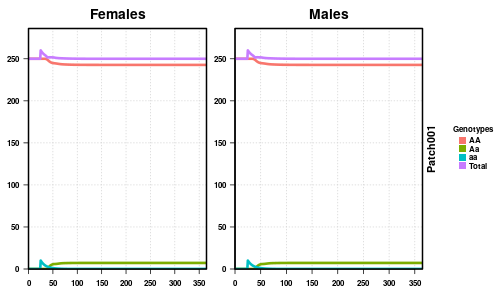In the figure, we see males and females of every genotype plotted by time, along with the total population. With an equilibrium population size of 500 individuals, and no sex biasing, we have an equal division of males and females. The release occurs equally in both sexes at time t = 25, as expected, and we see an increase in the total population due to the release before recovering to the equilibrium population size. Since this is basic Mendelian inheritance, we should be able to calculate the expected allele and genotype frequencies assuming Hardy-Weinberg equilibrium (HWE). As we released 20 aa individuals into a population of 500 AA individuals, we can calculate the expected allele frequencies: • a: $$\frac{1}{26}$$ • A: $$\frac{25}{26}$$ This leads to the following expected genotype frequencies: • AA: $$\big(\frac{25}{26}\big)^2 =$$ 0.9246 • Aa: $$2\cdot \big(\frac{25}{26}\big)\cdot\big(\frac{1}{26}\big) =$$ 0.0740 • aa: $$\big(\frac{1}{26}\big)^2 =$$ 0.0015 Which, in a population of size 500, implies the following number of individuals (remember, this is a deterministic simulation, so “individuals” will not be whole numbers): • AA: 462.28 • Aa: 36.98 • aa: 0.74 However, when we look at the equilibrium genotypes from the simulation, the populations are: • AA: 485.47 • Aa: 14.42 • aa: 0.11 These are significantly different from the expected genotype numbers according to HWE. This is because of the assumptions in how HWE is calculated, specifically, ignoring maturation of child stages prior to reproduction by assuming discrete generations, and infinite population size. In contrast, MGDrivE has overlapping generations and tracks offspring development prior to adulthood. Additionally, while the release performed here is small, the population is far from “infinite”, and the release represents 4% of the population. Therefore, the effective population size is significantly larger than 500. Ignoring the effect of constant daily mortality on the distribution of adults, implying individual lifespans follow a geometric distribution and reducing the proportion of released individuals to wild-type as adults die off, we can calculate the effective population size using the following parameters; • Adult Population Size: 500 • Release Amount: 20 • Daily Pupation Amount: 45 • Maturation Time: 15 days From these parameters, an approximate effective population size is calculated by combining the Adult Population Size, plus the Release Amount, plus the Maturation Time times the Daily Pupation Amount, which estimates a population size of 1195. Using this estimate of our effective population size, the expected allele frequencies are: • a: $$\frac{4}{239}$$ • A: $$\frac{235}{239}$$ Our new expected genotype frequencies: • AA: $$\big(\frac{235}{239}\big)^2 =$$ 0.96681 • Aa: $$2\cdot \big(\frac{235}{239}\big)\cdot\big(\frac{4}{239}\big) =$$ 0.03291 • aa: $$\big(\frac{4}{239}\big)^2 =$$ 0.00028 Since our population will return to equilibrium after some time, 500 individuals, we expect the following number of individuals of each genotype: • AA: 483.40 • Aa: 16.46 • aa: 0.14 This is significantly closer to the simulated population. As expected, it is slightly high, which stems from ignoring the geometric distribution of our adult population. #### Deterministic, With Fitness Cost Now that we have shown a basic Mendelian simulation, we will expand it a little by including a fitness cost on certain genotypes. In the following simulation, the homozygotes, AA and aa, are only 60% as fit as the heterozygote over their adult lifetime. This is an example of a heterozygote advantage, and we should see the population become primarily heterozygous. We will increase the size and number of releases and perform them a little bit later, to see the effects of the fitness cost and then speed up the equilibration process, but everything else will remain the same, i.e., one population and a deterministic simulation. #################### # Load libraries #################### library(MGDrivE) #################### # Output Folder #################### outFolder <- "mgdrive" #################### # Simulation Parameters #################### # days to run the simulation tMax <- 365*2 # entomological parameters bioParameters <- list(betaK=20, tEgg=5, tLarva=6, tPupa=4, popGrowth=1.175, muAd=0.09) # a 1-node network where mosquitoes do not leave moveMat <- as.matrix(1) # parameters of the population equilibrium adultPopEquilibrium <- 500 sitesNumber <- nrow(moveMat) patchPops <- rep(adultPopEquilibrium,sitesNumber) #################### # Basic Inheritance pattern #################### # Mendelian cube with standard (default) parameters # This time, lets put fitness cost on the homozygotes, giving the heterozygote # an advantage # These genotypes correspond to ones in the cube. Look at a base cube first, # then set this. # homozygotes are 60% as fit as heterozygote over their entire lifetime # Since omega is the adult daily death rate, we use the built-in function to # calculate our desired lifetime cost as applied daily dayOmega <- calcOmega(mu = bioParameters$muAd, lifespanReduction = 0.60)
omegaNew <- c("AA"=dayOmega, "aa"=dayOmega)

# setup cube
cube <- cubeMendelian(omega = omegaNew)

####################
# Setup releases and batch migration
####################
# set up the empty release vector
#  MGDrivE pulls things out by name
patchReleases <- replicate(n=sitesNumber,
expr={list(maleReleases=NULL,femaleReleases=NULL,
eggReleases=NULL,matedFemaleReleases=NULL)},
simplify=FALSE)

# choose release parameters
#  Releases start at time 100, occur every day, for 5 days.
#  There are 50 mosquitoes released every time.
releasesParameters <- list(releasesStart=100,
releasesNumber=5,
releasesInterval=0,
releaseProportion=50)

# generate male release vector
maleReleasesVector <- generateReleaseVector(driveCube=cube,
releasesParameters=releasesParameters)

# generate female release vector
femaleReleasesVector <- generateReleaseVector(driveCube=cube,
releasesParameters=releasesParameters)

# put releases into the proper place in the release list
patchReleases[]$maleReleases <- maleReleasesVector patchReleases[]$femaleReleases <- femaleReleasesVector

# batch migration is disabled by setting the probability to 0
# This is required because of the stochastic simulations, but doesn't make sense
#  in a deterministic simulation.
batchMigration <- basicBatchMigration(batchProbs=0,
sexProbs=c(.5,.5),
numPatches=sitesNumber)

####################
# Combine parameters and run!
####################
# set MGDrivE to run deterministic
setupMGDrivE(stochasticityON = FALSE, verbose = FALSE)

# setup parameters for the network. This builds a list of parameters required for
#  every population in the network. In ths case, we have a network of 1 population.
netPar <- parameterizeMGDrivE(runID=1, simTime=tMax, nPatch=sitesNumber,
beta=bioParameters$betaK, muAd=bioParameters$muAd,
popGrowth=bioParameters$popGrowth, tEgg=bioParameters$tEgg,
tLarva=bioParameters$tLarva, tPupa=bioParameters$tPupa,

# build network prior to run
MGDrivESim <- Network$new(params=netPar, driveCube=cube, patchReleases=patchReleases, migrationMale=moveMat, migrationFemale=moveMat, migrationBatch=batchMigration, directory=outFolder, verbose=FALSE) # run simulation MGDrivESim$oneRun(verbose = FALSE)

####################
# Post Analysis
####################
# split output by patch
#  Required for plotting later
splitOutput(readDir = outFolder, remFile = TRUE, verbose = FALSE)

# aggregate females by their mate choice
#  This reduces the female file to have the same columns as the male file
aggregateFemales(readDir = outFolder, genotypes = cube$genotypesID, remFile = TRUE, verbose = FALSE) # plot output to see effect plotMGDrivESingle(readDir = outFolder, totalPop = TRUE, lwd = 3.5, alpha = 1)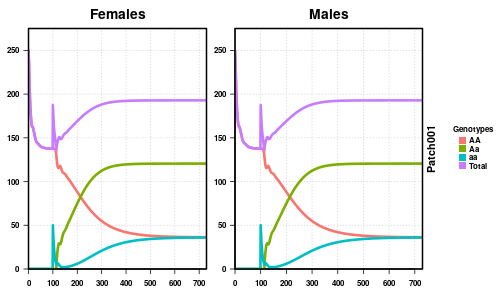Again, we plot the females on the left, the males on the right, with the population size on the Y-axis and simulation time along the X-axis. We see the total population size starts at 250 males and females, a total of 500 individuals, but quickly drops to 275.125446. This is because of the fitness cost applied to the AA individuals that make up the population. As we applied an 60% fitness cost, we expect the total population to be 60% of the desired population. 60% of 500 individuals, assuming HWE applies, is 300, which is close to the simulated population size prior to the releases. This discrepancy can be accounted for by the presence of density-dependence during larval maturation. After releases of aa individuals are performed, we see the population quickly drive towards heterozygous individuals, and the population size marginally recover. The population partially recovers because there is no fitness cost on the heterozygotes, but does not fully recover because heterozygotes create homozygotes at each generation. At equilibrium, given that heterozygotes produce homozygotes at a rate of 50% per generation, our expected genotype amounts (assuming all HWE assumptions apply) are: • AA: $$500 \cdot \frac{1}{4} \cdot 0.60 = 75$$ • Aa: $$500 \cdot \frac{1}{2} \cdot 1.00 = 250$$ • aa: $$500 \cdot \frac{1}{4} \cdot 0.60 = 75$$ • Total: AA + Aa + aa = 400 Checking at the end of our simulation, we find that the empirical genotype amounts are: • AA: 72.58 • Aa: 241.03 • aa: 72.02 • Total: 385.63 This closely matches our simulated population, differing because HWE assumes discrete generations. #### Stochastic The processes of birth, death, mating, and inheritance are inherently probabilistic, meaning that in order to understand the full spectrum of outcomes a model may produce, it is necessary to simulate from correctly-specified stochastic models. We show an example of the same modeled system here when the stochastic simulation is used; we run an ensemble of simulations, then plot the trajectories to give a sense of the expected variability in possible model trajectories. We now use the same simulation parameters as the previous example but run the stochastic version of the model. To view variability in sampled trajectories, we run 50 repetitions. For realistic applications, large ensembles of simulations (>100) should be run and statistically analyzed to characterize quantities of interest. #################### # Load libraries #################### library(MGDrivE) #################### # Output Folder #################### # directory # This is slightly obtuse for vignette building reasons # Really, all you need is a base directory, then the repetitions in folders inside that. # Here, our base directory is "mgdrive", and the repetition folders are "001","002", etc. # So, the final structure is "mgdrive/001","mgdrive/002", etc. outFolder <- "mgdrive" dir.create(path = outFolder) #################### # Simulation Parameters #################### # days to run the simulation tMax <- 365*2 # number of Monte Carlo iterations nRep <- 50 # each Monte Carlo iteration gets its own folder folderNames <- file.path(outFolder, formatC(x = 1:nRep, width = 3, format = "d", flag = "0")) # entomological parameters bioParameters <- list(betaK=20, tEgg=5, tLarva=6, tPupa=4, popGrowth=1.175, muAd=0.09) # a 1-node network where mosquitoes do not leave moveMat <- as.matrix(1) # parameters of the population equilibrium adultPopEquilibrium <- 500 sitesNumber <- nrow(moveMat) #################### # Basic Inheritance pattern #################### # Mendelian cube with standard (default) parameters # This time, lets put fitness cost on the homozygotes, giving the heterozygote # an advantage # These genotypes correspond to ones in the cube. Look at a base cube first, # then set this. # homozygotes are 60% as fit as heterozygote over their entire lifetime # Since omega is the adult daily death rate, we use the built-in function to # calculate our desired lifetime cost as applied daily dayOmega <- calcOmega(mu = bioParameters$muAd, lifespanReduction = 0.60)
omegaNew <- c("AA"=dayOmega, "aa"=dayOmega)

# setup cube
cube <- cubeMendelian(omega = omegaNew)

####################
# Setup releases and batch migration
####################
# set up the empty release vector
#  MGDrivE pulls things out by name
patchReleases <- replicate(n=sitesNumber,
expr={list(maleReleases=NULL,femaleReleases=NULL,
eggReleases=NULL,matedFemaleReleases=NULL)},
simplify=FALSE)

# choose release parameters
#  Releases start at time 100, occur every day, for 5 days.
#  There are 50 mosquitoes released every time.
releasesParameters <- list(releasesStart=100,
releasesNumber=5,
releasesInterval=0,
releaseProportion=50)

# generate male release vector
maleReleasesVector <- generateReleaseVector(driveCube=cube,
releasesParameters=releasesParameters)

# generate female release vector
femaleReleasesVector <- generateReleaseVector(driveCube=cube,
releasesParameters=releasesParameters)

# put releases into the proper place in the release list
patchReleases[]$maleReleases <- maleReleasesVector patchReleases[]$femaleReleases <- femaleReleasesVector

# batch migration is disabled by setting the probability to 0
# This is required because of the stochastic simulations, but doesn't make sense
#  in a deterministic simulation.
batchMigration <- basicBatchMigration(batchProbs=0,
sexProbs=c(.5,.5),
numPatches=sitesNumber)

####################
# Combine parameters and run!
####################
# set MGDrivE to run stochastic
setupMGDrivE(stochasticityON = TRUE, verbose = FALSE)

# setup parameters for the network. This builds a list of parameters required for
#  every population in the network. In ths case, we have a network of 1 population.
netPar <- parameterizeMGDrivE(runID=1, simTime=tMax, sampTime = 5, nPatch=sitesNumber,
beta=bioParameters$betaK, muAd=bioParameters$muAd,
popGrowth=bioParameters$popGrowth, tEgg=bioParameters$tEgg,
tLarva=bioParameters$tLarva, tPupa=bioParameters$tPupa,

# build network prior to run
MGDrivESim <- Network$new(params=netPar, driveCube=cube, patchReleases=patchReleases, migrationMale=moveMat, migrationFemale=moveMat, migrationBatch=batchMigration, directory=folderNames, verbose = FALSE) # run simulation MGDrivESim$multRun(verbose = FALSE)

####################
# Post Analysis
####################
# First, split output by patch
# Second, aggregate females by their mate choice
for(i in 1:nRep){
splitOutput(readDir = folderNames[i], remFile = TRUE, verbose = FALSE)
aggregateFemales(readDir = folderNames[i], genotypes = cube$genotypesID, remFile = TRUE, verbose = FALSE) } # plot output of first run to see effect plotMGDrivESingle(readDir = folderNames, totalPop = TRUE, lwd = 3.5, alpha = 1)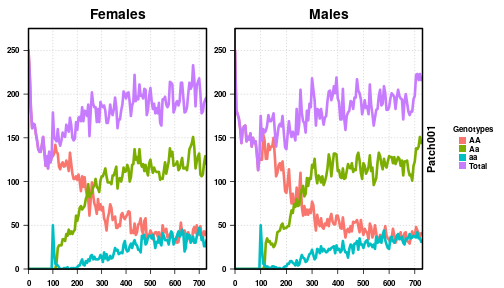# plot all 50 repetitions together plotMGDrivEMult(readDir = outFolder, lwd = 0.35, alpha = 0.75)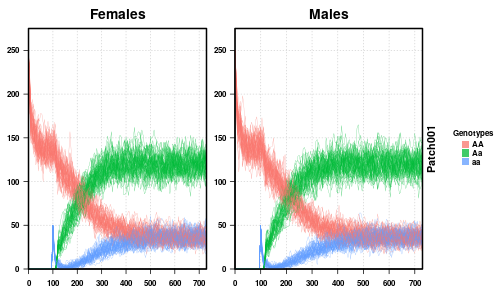We first plot a single run of our stochastic simulation, and then plot all 50 runs together. We see that the individual run looks similar to the deterministic run from before, all genotypes behaving heuristically the same. However, the dynamics are not as “clean” as the deterministic version, clearly suffering from the randomness inherent in an actual population, and the dynamics are slower than the deterministic version. This is an inherent effect of stochasticity, something that is important in decision making and with smaller population sizes. ### Mendelian Inheritance Simulations, Two Populations Single, panmictic populations are simple to analyze but rarely encountered in nature. Often, populations of identical individuals are separated by geography, be it great distances or a road that is difficult to cross. This creates structures within populations that can drastically alter how genes spread. Here, we explore how the results of our first simulation change when we have a structured population where two halves of the population never mix. MGDrivE handles population structure by considering separate, panmictic populations with some migration rate between them. This makes MGDrivE a meta-population model, where individual populations are part of the same graph, connected via some migration or mixture rate. In the following simulation, we use two nodes, or two well-mixed populations, that have no migration between them. Then, we simulate releases in one population, and analyze what happens. #### Deterministic, No Migration #################### # Load libraries #################### library(MGDrivE) #################### # Output Folder #################### outFolder <- "mgdrive" #################### # Simulation Parameters #################### # days to run the simulation tMax <- 365 # entomological parameters bioParameters <- list(betaK=20, tEgg=5, tLarva=6, tPupa=4, popGrowth=1.175, muAd=0.09) # a 2-node network where mosquitoes do not leave moveMat <- matrix(data = c(1,0,0,1), nrow = 2, ncol = 2) moveMat #> [,1] [,2] #> [1,] 1 0 #> [2,] 0 1 # parameters of the population equilibrium adultPopEquilibrium <- 500 sitesNumber <- nrow(moveMat) #################### # Basic Inheritance pattern #################### # Mendelian cube with standard (default) parameters cube <- cubeMendelian() #################### # Setup releases and batch migration #################### # set up the empty release vector # MGDrivE pulls things out by name patchReleases <- replicate(n=sitesNumber, expr={list(maleReleases=NULL,femaleReleases=NULL, eggReleases=NULL,matedFemaleReleases=NULL)}, simplify=FALSE) # choose release parameters # Releases start at time 25, occur every day, for 5 days. # There are 50 mosquitoes released every time. releasesParameters <- list(releasesStart=25, releasesNumber=5, releasesInterval=0, releaseProportion=50) # generate release vector releasesVector <- generateReleaseVector(driveCube=cube, releasesParameters=releasesParameters) # put releases into the proper place in the release list patchReleases[]$maleReleases <- releasesVector
patchReleases[]$femaleReleases <- releasesVector # batch migration is disabled by setting the probability to 0 # This is required because of the stochastic simulations, but doesn't make sense # in a deterministic simulation. batchMigration <- basicBatchMigration(batchProbs=0, sexProbs=c(.5,.5), numPatches=sitesNumber) #################### # Combine parameters and run! #################### # set MGDrivE to run deterministic setupMGDrivE(stochasticityON = FALSE, verbose = FALSE) # setup parameters for the network. This builds a list of parameters required for # every population in the network. In ths case, we have a network of 2 populations. netPar <- parameterizeMGDrivE(runID=1, simTime=tMax, nPatch=sitesNumber, beta=bioParameters$betaK, muAd=bioParameters$muAd, popGrowth=bioParameters$popGrowth, tEgg=bioParameters$tEgg, tLarva=bioParameters$tLarva, tPupa=bioParameters$tPupa, AdPopEQ=adultPopEquilibrium, inheritanceCube = cube) # build network prior to run MGDrivESim <- Network$new(params=netPar,
driveCube=cube,
patchReleases=patchReleases,
migrationMale=moveMat,
migrationFemale=moveMat,
migrationBatch=batchMigration,
directory=outFolder,
verbose=FALSE)
# run simulation
MGDrivESim$oneRun(verbose = FALSE) #################### # Post Analysis #################### # split output by patch # Required for plotting later splitOutput(readDir = outFolder, verbose = FALSE, remFile = TRUE) # aggregate females by their mate choice # This reduces the female file to have the same columns as the male file aggregateFemales(readDir = outFolder, genotypes = cube$genotypesID,
remFile = TRUE, verbose = FALSE)

# plot output to see effect
plotMGDrivESingle(readDir = outFolder, lwd = 3.5, alpha = 1)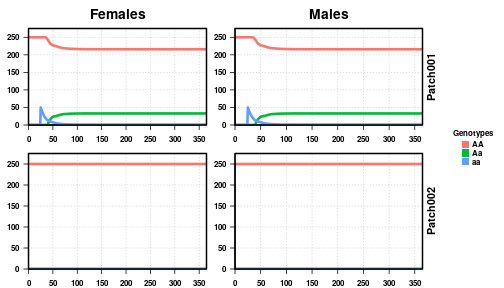The plots are now expanded to include both populations in our simulation, as labeled on the right side of the plot. We performed 5 releases of 50 aa individuals in the first node. However, since there is no migration between the populations, we see Aa individuals appear in patch 1 only, while patch 2 remains completely AA.

#### Deterministic, Small Migration

Now, if we increase the migration to 1% per day between the populations, we should see heterozygous individuals appear in patch 2, even though we only perform releases in patch 1. (We repeat the entire code for users' benefit.)

####################
####################
library(MGDrivE)

####################
# Output Folder
####################
outFolder <- "mgdrive"

####################
# Simulation Parameters
####################
# days to run the simulation
tMax <- 365

# entomological parameters
bioParameters <- list(betaK=20, tEgg=5, tLarva=6, tPupa=4, popGrowth=1.175, muAd=0.09)

# a 2-node network with 1% per day migration rate
moveMat <- matrix(data = c(0.99,0.01,0.01,0.99), nrow = 2, ncol = 2)
moveMat
#>      [,1] [,2]
#> [1,] 0.99 0.01
#> [2,] 0.01 0.99

# parameters of the population equilibrium
sitesNumber <- nrow(moveMat)

####################
# Basic Inheritance pattern
####################
# Mendelian cube with standard (default) parameters
cube <- cubeMendelian()

####################
# Setup releases and batch migration
####################
# set up the empty release vector
#  MGDrivE pulls things out by name
patchReleases <- replicate(n=sitesNumber,
expr={list(maleReleases=NULL,femaleReleases=NULL,
eggReleases=NULL,matedFemaleReleases=NULL)},
simplify=FALSE)

# choose release parameters
#  Releases start at time 25, occur every day, for 5 days.
#  There are 50 mosquitoes released every time.
releasesParameters <- list(releasesStart=25,
releasesNumber=5,
releasesInterval=0,
releaseProportion=50)

# generate male release vector
maleReleasesVector <- generateReleaseVector(driveCube=cube,
releasesParameters=releasesParameters)

# generate female release vector
femaleReleasesVector <- generateReleaseVector(driveCube=cube,
releasesParameters=releasesParameters)

# put releases into the proper place in the release list
patchReleases[]$maleReleases <- maleReleasesVector patchReleases[]$femaleReleases <- femaleReleasesVector

# batch migration is disabled by setting the probability to 0
# This is required because of the stochastic simulations, but doesn't make sense
#  in a deterministic simulation.
batchMigration <- basicBatchMigration(batchProbs=0,
sexProbs=c(.5,.5),
numPatches=sitesNumber)

####################
# Combine parameters and run!
####################
# set MGDrivE to run deterministic
setupMGDrivE(stochasticityON = FALSE, verbose = FALSE)

# setup parameters for the network. This builds a list of parameters required for
#  every population in the network. In ths case, we have a network of 2 populations.
netPar <- parameterizeMGDrivE(runID=1, simTime=tMax, nPatch=sitesNumber,
beta=bioParameters$betaK, muAd=bioParameters$muAd,
popGrowth=bioParameters$popGrowth, tEgg=bioParameters$tEgg,
tLarva=bioParameters$tLarva, tPupa=bioParameters$tPupa,

# build network prior to run
MGDrivESim <- Network$new(params=netPar, driveCube=cube, patchReleases=patchReleases, migrationMale=moveMat, migrationFemale=moveMat, migrationBatch=batchMigration, directory=outFolder, verbose = FALSE) # run simulation MGDrivESim$oneRun(verbose = FALSE)

####################
# Post Analysis
####################
# split output by patch
#  Required for plotting later
splitOutput(readDir = outFolder, verbose = FALSE, remFile = TRUE)

# aggregate females by their mate choice
#  This reduces the female file to have the same columns as the male file
aggregateFemales(readDir = outFolder, genotypes = cube$genotypesID, remFile = TRUE, verbose = FALSE) # plot output to see effect plotMGDrivESingle(readDir = outFolder, totalPop = TRUE, lwd = 3.5, alpha = 1)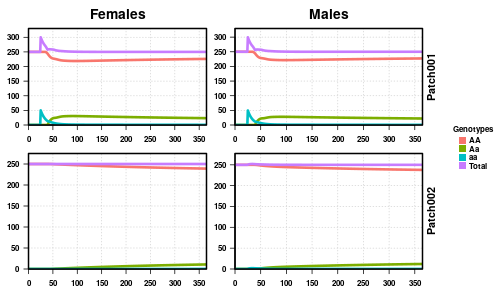As expected, with a 1% daily migration rate, we see heterozygotes in patch 2 shortly after they begin emerging in patch 1. #### Stochastic, Small Migration With very small migration rates, it is interesting to see how a scenario changes using a stochastic simulation. Some repetitions should take significantly longer for heterozygotes to migrate from patch 1 to patch 2. So, we keep all of the parameters the same as above, but run 25 stochastic repetitions instead of a deterministic simulation. #################### # Load libraries #################### library(MGDrivE) #################### # Output Folder #################### # This is slightly obtuse for vignette building reasons # Really, all you need is a base directory, then the repetitions in folders inside that. # Here, our base directory is "mgdrive", and the repetition folders are "001","002", etc. # So, the final structure is "mgdrive/001","mgdrive/002", etc. outFolder <- "mgdrive" dir.create(path = outFolder) #################### # Simulation Parameters #################### # days to run the simulation tMax <- 365 # number of Monte Carlo iterations nRep <- 25 # each Monte Carlo iteration gets its own folder folderNames <- file.path(outFolder, formatC(x = 1:nRep, width = 3, format = "d", flag = "0")) # entomological parameters bioParameters <- list(betaK=20, tEgg=5, tLarva=6, tPupa=4, popGrowth=1.175, muAd=0.09) # a 2-node network with 1% per day migration rate moveMat <- matrix(data = c(0.99,0.01,0.01,0.99), nrow = 2, ncol = 2) moveMat #> [,1] [,2] #> [1,] 0.99 0.01 #> [2,] 0.01 0.99 # parameters of the population equilibrium adultPopEquilibrium <- 500 sitesNumber <- nrow(moveMat) #################### # Basic Inheritance pattern #################### # Mendelian cube with standard (default) parameters cube <- cubeMendelian() #################### # Setup releases and batch migration #################### # set up the empty release vector # MGDrivE pulls things out by name patchReleases <- replicate(n=sitesNumber, expr={list(maleReleases=NULL,femaleReleases=NULL, eggReleases=NULL,matedFemaleReleases=NULL)}, simplify=FALSE) # choose release parameters # Releases start at time 25, occur every day, for 5 days. # There are 50 mosquitoes released every time. releasesParameters <- list(releasesStart=25, releasesNumber=5, releasesInterval=0, releaseProportion=50) # generate release vector releasesVector <- generateReleaseVector(driveCube=cube, releasesParameters=releasesParameters) # put releases into the proper place in the release list patchReleases[]$maleReleases <- releasesVector
patchReleases[]$femaleReleases <- releasesVector # batch migration is disabled by setting the probability to 0 # This is required because of the stochastic simulations, but doesn't make sense # in a deterministic simulation. batchMigration <- basicBatchMigration(batchProbs=0, sexProbs=c(.5,.5), numPatches=sitesNumber) #################### # Combine parameters and run! #################### # set MGDrivE to run stochastic setupMGDrivE(stochasticityON = TRUE, verbose = FALSE) # setup parameters for the network. This builds a list of parameters required for # every population in the network. In ths case, we have a network of 2 populations. netPar <- parameterizeMGDrivE(runID=1, simTime=tMax, nPatch=sitesNumber, beta=bioParameters$betaK, muAd=bioParameters$muAd, popGrowth=bioParameters$popGrowth, tEgg=bioParameters$tEgg, tLarva=bioParameters$tLarva, tPupa=bioParameters$tPupa, AdPopEQ=adultPopEquilibrium, inheritanceCube = cube) # build network prior to run MGDrivESim <- Network$new(params=netPar,
driveCube=cube,
patchReleases=patchReleases,
migrationMale=moveMat,
migrationFemale=moveMat,
migrationBatch=batchMigration,
directory=folderNames,
verbose = FALSE)
# run simulation
MGDrivESim$multRun(verbose = FALSE) #################### # Post Analysis #################### # First, split output by patch # Second, aggregate females by their mate choice for(i in 1:nRep){ splitOutput(readDir = folderNames[i], remFile = TRUE, verbose = FALSE) aggregateFemales(readDir = folderNames[i], genotypes = cube$genotypesID,
remFile = TRUE, verbose = FALSE)
}

# plot output of first run to see effect
plotMGDrivESingle(readDir = folderNames, totalPop = TRUE, lwd = 3.5, alpha = 1)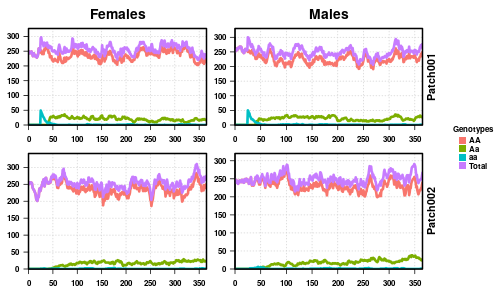# plot all 25 repetitions together
plotMGDrivEMult(readDir = outFolder, lwd = 0.35, alpha = 0.75)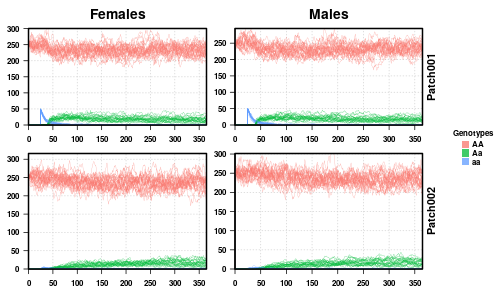Looking at the first plot, of just one repetition from the 25 that we ran, we see the release at time 25 and then the increase in heterozygotes after that. If we look closely, there are a few aa individuals that migrate to the second patch, but very few. We see how the population of Aa individuals fluctuates near zero until the end of the simulation, but there are enough that they never completely drop out of the population. The total population fluctuates around 500 individuals, 250 each of males and females, as expected from the parameters provided.

Looking at the second plot with all 25 repetitions provided, we see how the simulations heuristically agree with the deterministic ones performed above. The general analysis remains the same here, but with more noise, accounting for the random fluctuations in real populations. While not particularly enlightening in this setting, the behavior and outcome are the same, it's easy to see how low-frequency genotypes could be lost from a population.

#### Stochastic, Small Migration and Fitness Cost

As a final example, we perform a stochastic, two-population simulation with the same fitness cost explored previously, i.e., a 60% reduction in lifetime on AA and aa individuals. We first explored this in a single, panmictic population using a deterministic simulation, then explored the effect of stochasticity on the results. Here, we extend our analysis to a two-population setting, to explore how population structure might affect our results.

####################
####################
library(MGDrivE)

####################
# Output Folder
####################
# This is slightly obtuse for vignette building reasons
#  Really, all you need is a base directory, then the repetitions in folders inside that.
#  Here, our base directory is "mgdrive", and the repetition folders are "001","002", etc.
#  So, the final structure is "mgdrive/001","mgdrive/002", etc.
outFolder <- "mgdrive"
dir.create(path = outFolder)

####################
# Simulation Parameters
####################
# days to run the simulation
tMax <- 365*2

# number of Monte Carlo iterations
nRep <- 25

# each Monte Carlo iteration gets its own folder
folderNames <- file.path(outFolder,
formatC(x = 1:nRep, width = 3, format = "d", flag = "0"))

# entomological parameters
bioParameters <- list(betaK=20, tEgg=5, tLarva=6, tPupa=4, popGrowth=1.175, muAd=0.09)

# a 2-node network with 1% per day migration rate
moveMat <- matrix(data = c(0.99,0.01,0.01,0.99), nrow = 2, ncol = 2)

# parameters of the population equilibrium
sitesNumber <- nrow(moveMat)

####################
# Basic Inheritance pattern
####################
# Mendelian cube

# This time, lets put fitness cost on the homozygotes, giving the heterozygote

# These genotypes correspond to ones in the cube. Look at a base cube first,
#  then set this.
# Homozygotes are 60% as fit as heterozygote over their entire lifetime
#  Since omega is a daily death rate, we use the built-in function to calculate
#  our desired lifetime cost as applied daily
dayOmega <- calcOmega(mu = bioParameters$muAd, lifespanReduction = 0.60) omegaNew <- c("AA"=dayOmega, "aa"=dayOmega) # setup cube cube <- cubeMendelian(omega = omegaNew) #################### # Setup releases and batch migration #################### # set up the empty release vector # MGDrivE pulls things out by name patchReleases <- replicate(n=sitesNumber, expr={list(maleReleases=NULL,femaleReleases=NULL, eggReleases=NULL,matedFemaleReleases=NULL)}, simplify=FALSE) # choose release parameters # Releases start at time 100, occur every day, for 5 days. # There are 50 mosquitoes released every time. releasesParameters <- list(releasesStart=100, releasesNumber=5, releasesInterval=0, releaseProportion=50) # generate male release vector maleReleasesVector <- generateReleaseVector(driveCube=cube, releasesParameters=releasesParameters) # generate female release vector femaleReleasesVector <- generateReleaseVector(driveCube=cube, releasesParameters=releasesParameters) # put releases into the proper place in the release list patchReleases[]$maleReleases <- maleReleasesVector
patchReleases[]$femaleReleases <- femaleReleasesVector # batch migration is disabled by setting the probability to 0 # This is required because of the stochastic simulations, but doesn't make sense # in a deterministic simulation. batchMigration <- basicBatchMigration(batchProbs=0, sexProbs=c(.5,.5), numPatches=sitesNumber) #################### # Combine parameters and run! #################### # set MGDrivE to run stochastic setupMGDrivE(stochasticityON = TRUE, verbose = FALSE) # setup parameters for the network. This builds a list of parameters required for # every population in the network. In ths case, we have a network of 2 populations. netPar <- parameterizeMGDrivE(runID=1, simTime=tMax, nPatch=sitesNumber, beta=bioParameters$betaK, muAd=bioParameters$muAd, popGrowth=bioParameters$popGrowth, tEgg=bioParameters$tEgg, tLarva=bioParameters$tLarva, tPupa=bioParameters$tPupa, AdPopEQ=adultPopEquilibrium, inheritanceCube = cube) # build network prior to run MGDrivESim <- Network$new(params=netPar,
driveCube=cube,
patchReleases=patchReleases,
migrationMale=moveMat,
migrationFemale=moveMat,
migrationBatch=batchMigration,
directory=folderNames,
verbose = FALSE)
# run simulation
MGDrivESim$multRun(verbose = FALSE) #################### # Post Analysis #################### # First, split output by patch # Second, aggregate females by their mate choice for(i in 1:nRep){ splitOutput(readDir = folderNames[i], remFile = TRUE, verbose = FALSE) aggregateFemales(readDir = folderNames[i], genotypes = cube$genotypesID,
remFile = TRUE, verbose = FALSE)
}

# plot output of first run to see effect
#  per the structure above, we are reading "mgdrive/001" for the single plot
plotMGDrivESingle(readDir = folderNames, totalPop = TRUE, lwd = 3.5, alpha = 1)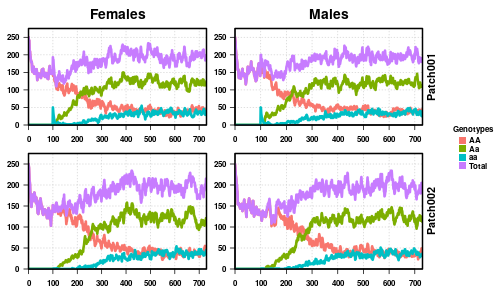# plot all 50 repetitions together
#  Here, we feed the function "mgdrive/", and it finds all repetition folders
#   inside that.
plotMGDrivEMult(readDir = outFolder, lwd = 0.35, alpha = 0.75)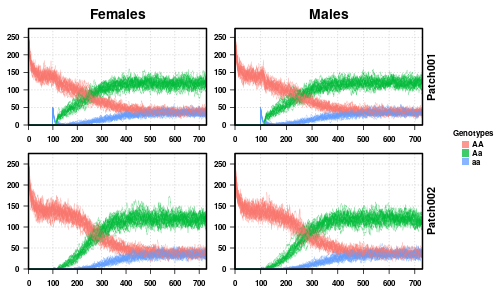Looking at the first plot, a single repetition of our stochastic simulation, we see that the results are very similar. The population size first drops to about 300, 60% of the equilibration population, and then increases to about 400, as expected from the deterministic simulation and analysis performed before. Do notice however, the dynamics are slower in the first population than in the single-population exploration. This is because we performed the same releases, but with a second population, there is a small immigration of AA individuals into the first patch and a small emigration of aa and Aa individuals out of patch 1 into patch 2. Thus, there is effectively twice the population size total, even though there is only a 1% chance of mixing per day.

The second plot shows all 25 repetitions of our simulation. The results clearly follow the one-node, deterministic simulation performed before. There is an initial population drop, as both patches are fully AA until we perform releases, then releases and a small recovery in population size. The general dynamics remain very similar, with the shift from AA to Aa individuals in the population taking a little longer than originally. This is also something we saw in the one-node stochastic simulation, and is a result of stochasticity in the population dynamics, as well as migration to/from the second patch.

### Reciprocal Translocation Gene Drive Simulations, One Population

Reciprocal translocations are a classic form of gene drive, utilizing the underdominance effects of gene dosage compensation to either suppress a population or replace a population, depending on fitness costs and release frequencies. The classic reciprocal translocation involves breaking two chromosomes, swapping the broken ends, and reattaching the ends to the opposite chromosomes. Theoretically, this creates a perfect 50% fitness cost on the population, as has been shown previously. However, previous simulations studying reciprocal translocations have been panmictic, deterministic, and ignore life-stages. Thus, we start with two deterministic simulations, to find the critical threshold when a complete life-history is included, and then explore how that threshold is affected by stochasticity.

#### Deterministic, Below Threshold

We maintain the same theoretical fitness cost, namely, that possession of only 1 copy of a reciprocal chromosome is always fatal. We perform 5 releases of transgenic critters, both male and female, every 7 days starting at day 25. This is not enough to make the population 50% transgenic, and therefore the transgenic critters should die out.

####################
####################
library(MGDrivE)

####################
# Output Folder
####################
outFolder <- "mgdrive"

####################
# Simulation Parameters
####################
# days to run the simulation, 2 years
tMax <- 365*2

# entomological parameters
bioParameters <- list(betaK=20, tEgg=5, tLarva=6, tPupa=4, popGrowth=1.175, muAd=0.09)

# a 1-node network where mosquitoes do not leave
moveMat <- as.matrix(1)

# parameters of the population equilibrium
sitesNumber <- nrow(moveMat)

####################
# Basic Inheritance pattern
####################
# Reciprocal translocation cube with standard (default) parameters
cube <- cubeReciprocalTranslocations()

####################
# Setup releases and batch migration
####################
# set up the empty release vector
#  MGDrivE pulls things out by name
patchReleases <- replicate(n=sitesNumber,
expr={list(maleReleases=NULL,femaleReleases=NULL,
eggReleases=NULL,matedFemaleReleases=NULL)},
simplify=FALSE)

# choose release parameters
#  Releases start at time 25, occur once a week, for 5 weeks.
#  There are 100 mosquitoes released every time.
releasesParameters <- list(releasesStart=25,
releasesNumber=5,
releasesInterval=7,
releaseProportion=100)

# generate male release vector
maleReleasesVector <- generateReleaseVector(driveCube=cube,
releasesParameters=releasesParameters)

# generate female release vector
femaleReleasesVector <- generateReleaseVector(driveCube=cube,
releasesParameters=releasesParameters)

# put releases into the proper place in the release list
patchReleases[]$maleReleases <- maleReleasesVector patchReleases[]$femaleReleases <- femaleReleasesVector

# batch migration is disabled by setting the probability to 0
# This is required because of the stochastic simulations, but doesn't make sense
#  in a deterministic simulation.
batchMigration <- basicBatchMigration(batchProbs=0,
sexProbs=c(.5,.5),
numPatches=sitesNumber)

####################
# Combine parameters and run!
####################
# set MGDrivE to run deterministic
setupMGDrivE(stochasticityON = FALSE, verbose = FALSE)

# setup parameters for the network. This builds a list of parameters required for
#  every population in the network. In ths case, we have a network of 1 population.
netPar <- parameterizeMGDrivE(runID=1, simTime=tMax, nPatch=sitesNumber,
beta=bioParameters$betaK, muAd=bioParameters$muAd,
popGrowth=bioParameters$popGrowth, tEgg=bioParameters$tEgg,
tLarva=bioParameters$tLarva, tPupa=bioParameters$tPupa,

# build network prior to run
MGDrivESim <- Network$new(params=netPar, driveCube=cube, patchReleases=patchReleases, migrationMale=moveMat, migrationFemale=moveMat, migrationBatch=batchMigration, directory=outFolder, verbose = FALSE) # run simulation MGDrivESim$oneRun(verbose = FALSE)

####################
# Post Analysis
####################
# split output by patch
#  Required for plotting later
splitOutput(readDir = outFolder, remFile = TRUE, verbose = FALSE)

# aggregate females by their mate choice
#  This reduces the female file to have the same columns as the male file
aggregateFemales(readDir = outFolder, genotypes = cube$genotypesID, remFile = TRUE, verbose = FALSE) # plot output to see effect plotMGDrivESingle(readDir = outFolder, totalPop = TRUE, lwd = 3.5, alpha = 1)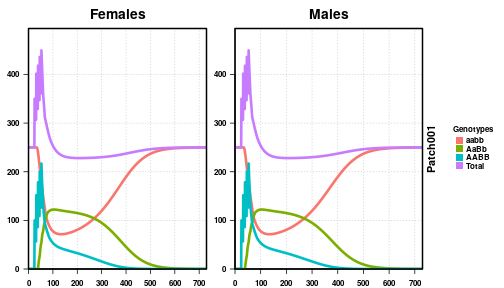We plot the number of female (left) and male (right) critters over a period of two years. The total population is shown in purple, while wild-type (aabb) individuals are in orange and fully transgenic individuals (AABB) are in light blue. We see the total population increase dramatically during the releases, then fall back to a little below the equilibrium amount. This is because of offspring of transgenic and wild-type critters than are non-viable. Eventually, the transgenic individuals completely die out and the population is wild-type again. This is the expected behavior for reciprocal translocations when the 50% critical threshold is not achieved. #### Deterministic, Above Threshold Now, we perform the same simulation as above, but increase the number of releases by one, from 5 releases to 6. This is enough to get over the critical threshold, so we should see the population turn entirely into transgenic individuals. #################### # Load libraries #################### library(MGDrivE) #################### # Output Folder #################### outFolder <- "mgdrive" #################### # Simulation Parameters #################### # days to run the simulation, 2 years tMax <- 365*2 # entomological parameters bioParameters <- list(betaK=20, tEgg=5, tLarva=6, tPupa=4, popGrowth=1.175, muAd=0.09) # a 1-node network where mosquitoes do not leave moveMat <- as.matrix(1) # parameters of the population equilibrium adultPopEquilibrium <- 500 sitesNumber <- nrow(moveMat) patchPops <- rep(adultPopEquilibrium,sitesNumber) #################### # Basic Inheritance pattern #################### # Reciprocal translocation cube with standard (default) parameters cube <- cubeReciprocalTranslocations() #################### # Setup releases and batch migration #################### # set up the empty release vector # MGDrivE pulls things out by name patchReleases <- replicate(n=sitesNumber, expr={list(maleReleases=NULL,femaleReleases=NULL, eggReleases=NULL,matedFemaleReleases=NULL)}, simplify=FALSE) # choose release parameters # Releases start at time 25, occur once a week, for 6 weeks. # There are 100 mosquitoes released every time. releasesParameters <- list(releasesStart=25, releasesNumber=6, releasesInterval=7, releaseProportion=100) # generate release vector releasesVector <- generateReleaseVector(driveCube=cube, releasesParameters=releasesParameters) # put releases into the proper place in the release list patchReleases[]$maleReleases <- releasesVector
patchReleases[]$femaleReleases <- releasesVector # batch migration is disabled by setting the probability to 0 # This is required because of the stochastic simulations, but doesn't make sense # in a deterministic simulation. batchMigration <- basicBatchMigration(batchProbs=0, sexProbs=c(.5,.5), numPatches=sitesNumber) #################### # Combine parameters and run! #################### # set MGDrivE to run deterministic setupMGDrivE(stochasticityON = FALSE, verbose = FALSE) # setup parameters for the network. This builds a list of parameters required for # every population in the network. In ths case, we have a network of 1 population. netPar <- parameterizeMGDrivE(runID=1, simTime=tMax, nPatch=sitesNumber, beta=bioParameters$betaK, muAd=bioParameters$muAd, popGrowth=bioParameters$popGrowth, tEgg=bioParameters$tEgg, tLarva=bioParameters$tLarva, tPupa=bioParameters$tPupa, AdPopEQ=patchPops, inheritanceCube = cube) # build network prior to run MGDrivESim <- Network$new(params=netPar,
driveCube=cube,
patchReleases=patchReleases,
migrationMale=moveMat,
migrationFemale=moveMat,
migrationBatch=batchMigration,
directory=outFolder,
verbose = FALSE)
# run simulation
MGDrivESim$oneRun(verbose = FALSE) #################### # Post Analysis #################### # split output by patch # Required for plotting later splitOutput(readDir = outFolder, remFile = TRUE, verbose = FALSE) # aggregate females by their mate choice # This reduces the female file to have the same columns as the male file aggregateFemales(readDir = outFolder, genotypes = cube$genotypesID,
remFile = TRUE, verbose = FALSE)

# plot output to see effect
plotMGDrivESingle(readDir = outFolder, totalPop = TRUE, lwd = 3.5, alpha = 1)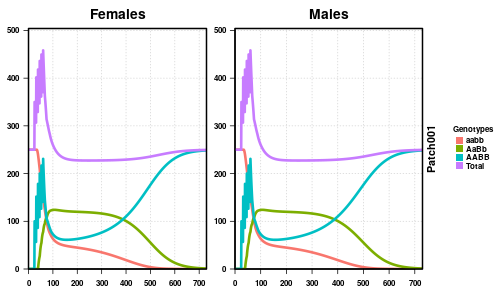Analyzing the same plots as above, notice one more release and the dramatic change it has on the outcome of the simulation. This time, our releases surpassed the critical threshold and the transgenic critters took over the population. Again though, we see the slight depression in population size initially after releases, while the population is a mix of wild-type and transgenics. However, once the population is entirely transgenic, the total size returns to the equilibrium value.

#### Stochastic, “Above” Threshold

The preceding two simulations show dramatically different results with the addition of a single release. This implies that we are near the critical threshold but gives a false impression of how distinct or certain we are of the outcome. Stochastic fluctuations can quickly push us across a threshold, even when deterministic simulations implied we had passed/not passed it. Using stochastic simulations, we can put a probability on an outcome, given that we are near the threshold. Thus, we perform the same simulation as above, 6 releases, that we think puts us over the critical threshold. However, as we will see below, this is not entirely the case.

####################
####################
library(MGDrivE)

####################
# Output Folder
####################
# This is slightly obtuse for vignette building reasons
#  Really, all you need is a base directory, then the repetitions in folders inside that.
#  Here, our base directory is "mgdrive", and the repetition folders are "001","002", etc.
#  So, the final structure is "mgdrive/001","mgdrive/002", etc.
outFolder <- "mgdrive"
dir.create(path = outFolder)

####################
# Simulation Parameters
####################
# days to run the simulation, 3 years
tMax <- 365*3

# number of Monte Carlo iterations
nRep <- 20

# each Monte Carlo iteration gets its own folder
folderNames <- file.path(outFolder,
formatC(x = 1:nRep, width = 3, format = "d", flag = "0"))

# entomological parameters
bioParameters <- list(betaK=20, tEgg=5, tLarva=6, tPupa=4, popGrowth=1.175, muAd=0.09)

# a 1-node network where mosquitoes do not leave
moveMat <- as.matrix(1)

# parameters of the population equilibrium
sitesNumber <- nrow(moveMat)

####################
# Basic Inheritance pattern
####################
# Reciprocal translocation cube with standard (default) parameters
cube <- cubeReciprocalTranslocations()

####################
# Setup releases and batch migration
####################
# set up the empty release vector
#  MGDrivE pulls things out by name
patchReleases <- replicate(n=sitesNumber,
expr={list(maleReleases=NULL,femaleReleases=NULL,
eggReleases=NULL,matedFemaleReleases=NULL)},
simplify=FALSE)

# choose release parameters
#  Releases start at time 25, occur once a week, for 6 weeks.
#  There are 100 mosquitoes released every time.
releasesParameters <- list(releasesStart=25,
releasesNumber=6,
releasesInterval=7,
releaseProportion=100)

# generate male release vector
maleReleasesVector <- generateReleaseVector(driveCube=cube,
releasesParameters=releasesParameters)

# generate female release vector
femaleReleasesVector <- generateReleaseVector(driveCube=cube,
releasesParameters=releasesParameters)

# put releases into the proper place in the release list
patchReleases[]$maleReleases <- maleReleasesVector patchReleases[]$femaleReleases <- femaleReleasesVector

# batch migration is disabled by setting the probability to 0
# This is required because of the stochastic simulations, but doesn't make sense
#  in a deterministic simulation.
batchMigration <- basicBatchMigration(batchProbs=0,
sexProbs=c(.5,.5),
numPatches=sitesNumber)

####################
# Combine parameters and run!
####################
# set MGDrivE to run stochastic
setupMGDrivE(stochasticityON = TRUE, verbose = FALSE)

# setup parameters for the network. This builds a list of parameters required for
#  every population in the network. In ths case, we have a network of 1 population.
netPar <- parameterizeMGDrivE(runID=1, simTime=tMax, nPatch=sitesNumber,
beta=bioParameters$betaK, muAd=bioParameters$muAd,
popGrowth=bioParameters$popGrowth, tEgg=bioParameters$tEgg,
tLarva=bioParameters$tLarva, tPupa=bioParameters$tPupa,

# build network prior to run
MGDrivESim <- Network$new(params=netPar, driveCube=cube, patchReleases=patchReleases, migrationMale=moveMat, migrationFemale=moveMat, migrationBatch=batchMigration, directory=folderNames, verbose = TRUE) #> initializing patch: 1 of 1 # run simulation MGDrivESim$multRun(verbose = FALSE)

####################
# Post Analysis
####################
# First, split output by patch
# Second, aggregate females by their mate choice
for(i in 1:nRep){
splitOutput(readDir = folderNames[i], remFile = TRUE, verbose = FALSE)
aggregateFemales(readDir = folderNames[i], genotypes = cube\$genotypesID,
remFile = TRUE, verbose = FALSE)
}

# plot output of first run to see effect
plotMGDrivESingle(readDir = folderNames, lwd = 3.5, alpha = 1)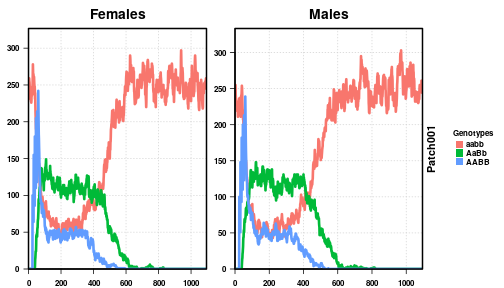# plot all 50 repetitions together
plotMGDrivEMult(readDir = outFolder, lwd = 0.35, alpha = 0.75)